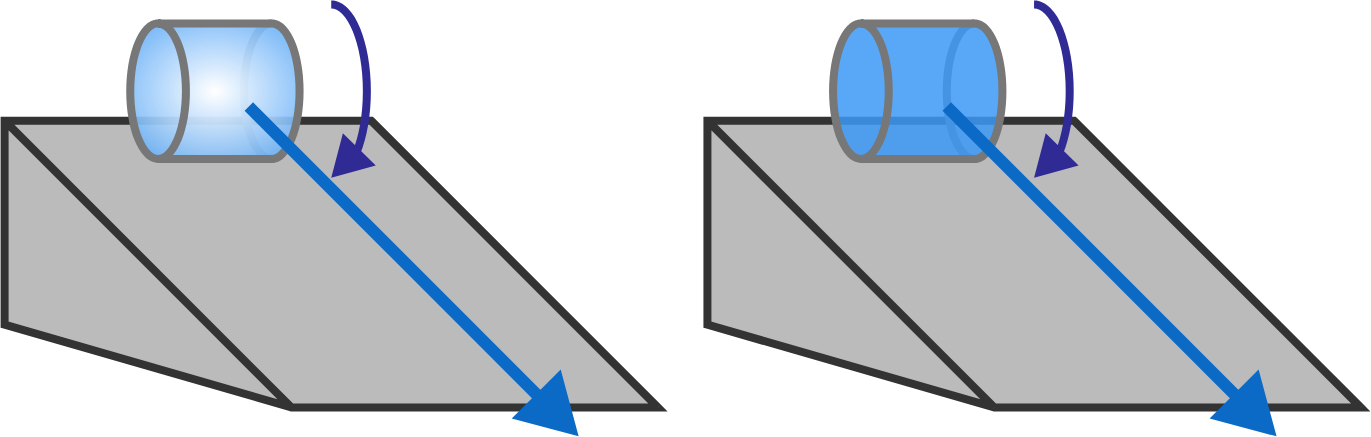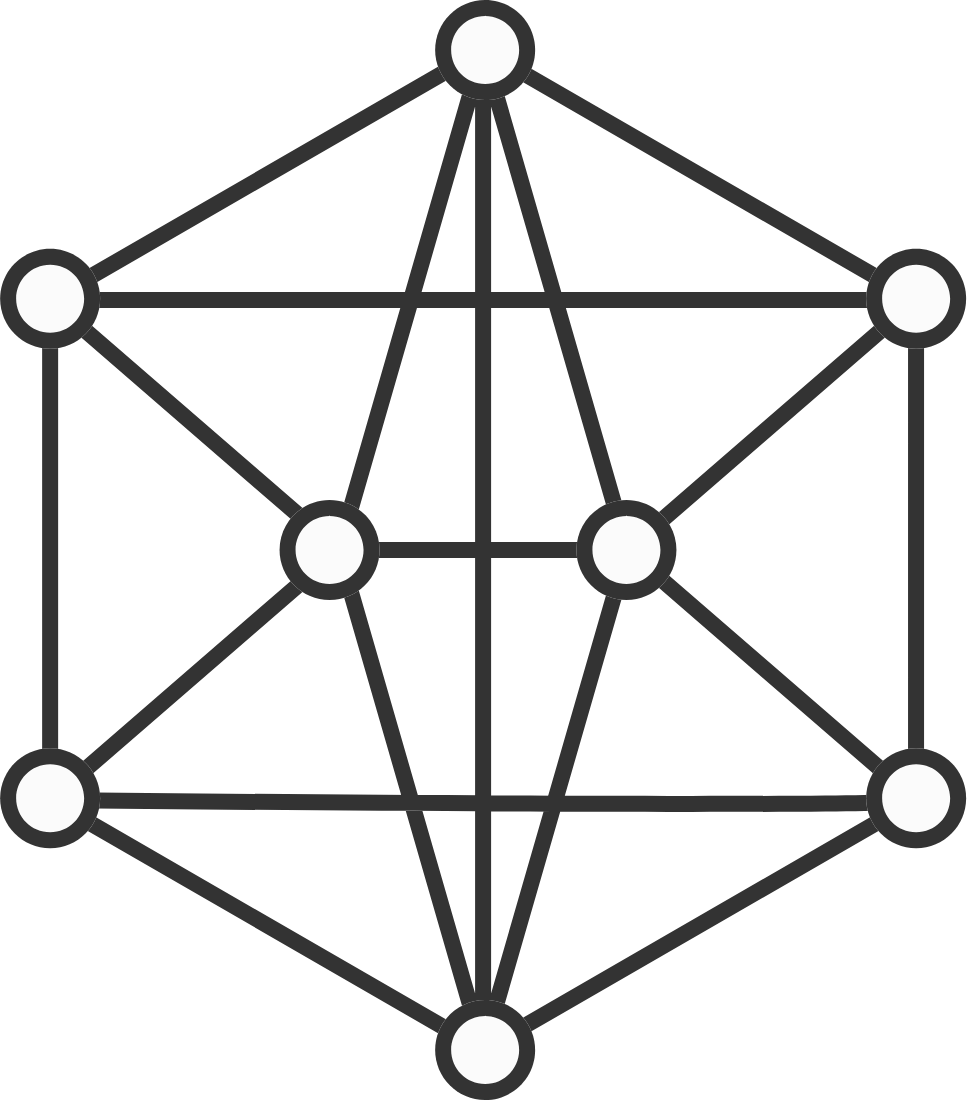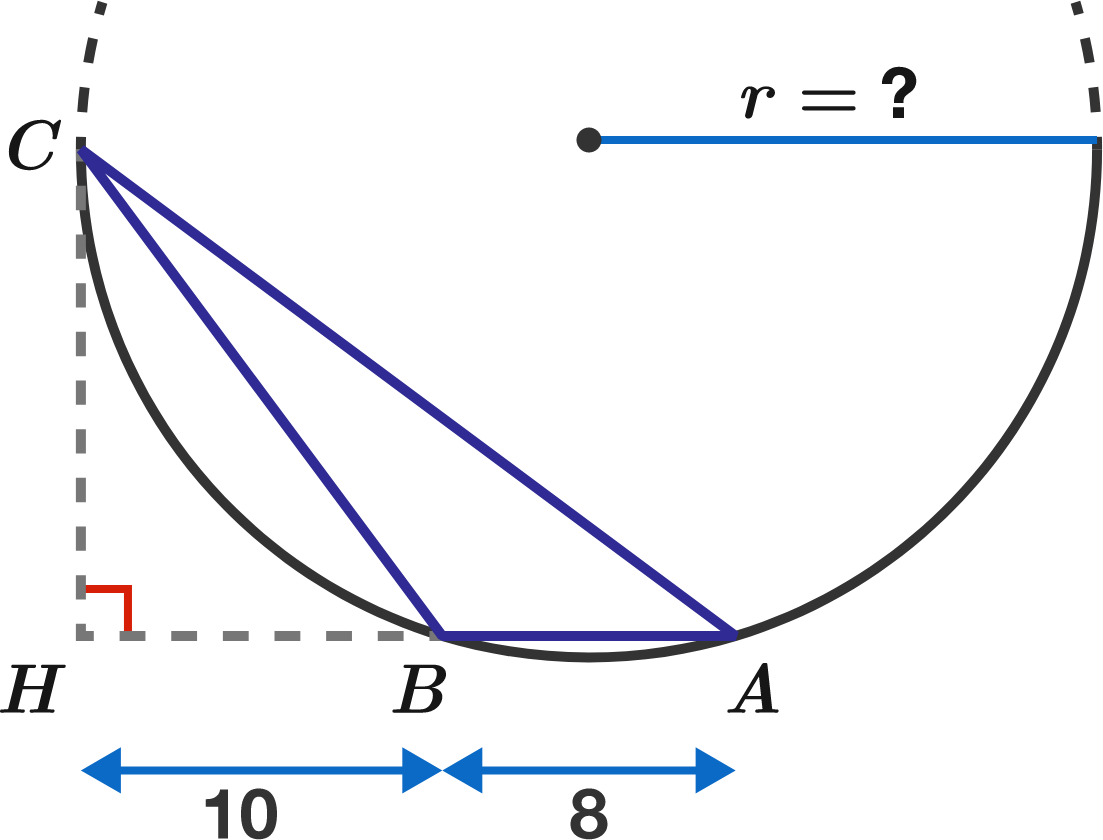# Problems of the Week

Contribute a problem

# 2018-04-02 Basic

A hollow cylinder is completely filled with frozen water.

Will it take longer to roll down an inclined plane while it’s frozen, or after it melts?Assume: When frozen, the water does not move relative to the cylinder.

What is the fewest number of colors needed such that no two adjacent vertices are colored the same?Note: In graph theory, two vertices are adjacent if they share an edge.

$\pm1\pm2\pm3\pm \cdots \pm2017\pm2018 = \, ?$

Using appropriate + and - signs on the left side of the equation, which number can be obtained on the right?Triangle $ABC$ is inscribed in a circle. The angle $ABC$ is obtuse, and the altitude $CH$ is tangent to the circle.

If $HB=10$ and $BA=8,$ what is the radius of the circle?

Tom was born on a Thursday in either 2014, 2015, 2016, or 2017. What is the oldest age Tom can turn on his next Thursday birthday?

Hint: Consider the remainder when the number of days in a year (365) is divided by the number of days in a week (7). But don't forget about leap years!

×